# Chemical Quantities Chapter 10 1 Molar Mass Molar

• Slides: 28Chemical Quantities Chapter 10 1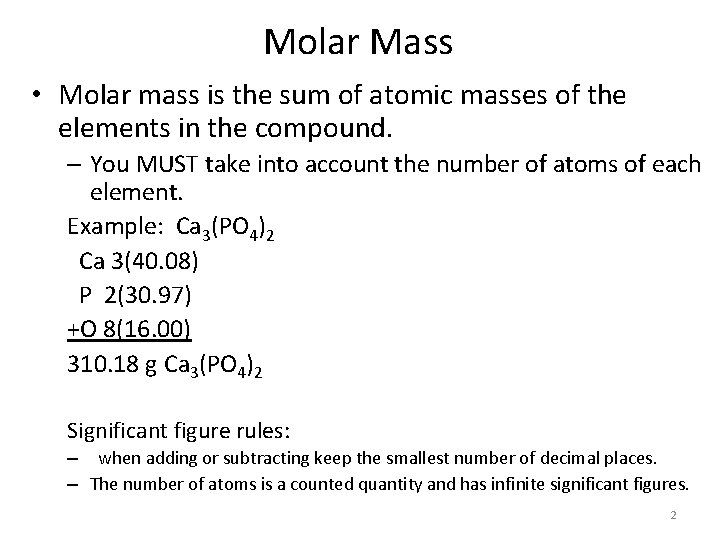Molar Mass • Molar mass is the sum of atomic masses of the elements in the compound. – You MUST take into account the number of atoms of each element. Example: Ca 3(PO 4)2 Ca 3(40. 08) P 2(30. 97) +O 8(16. 00) 310. 18 g Ca 3(PO 4)2 Significant figure rules: – when adding or subtracting keep the smallest number of decimal places. – The number of atoms is a counted quantity and has infinite significant figures. 2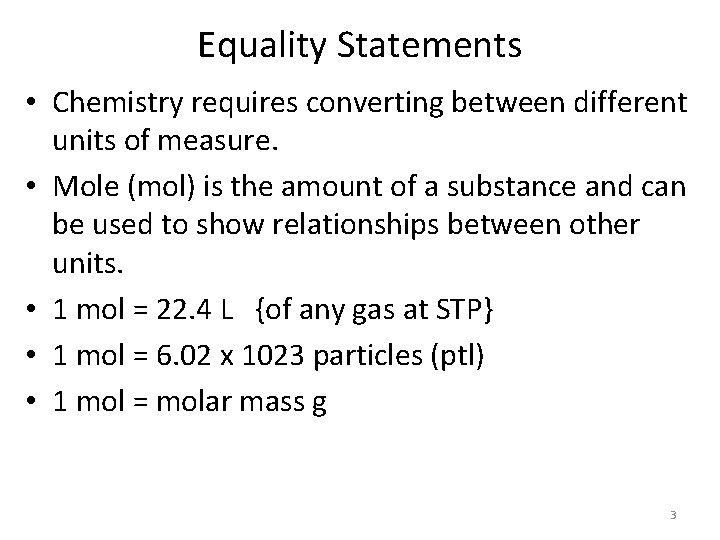Equality Statements • Chemistry requires converting between different units of measure. • Mole (mol) is the amount of a substance and can be used to show relationships between other units. • 1 mol = 22. 4 L {of any gas at STP} • 1 mol = 6. 02 x 1023 particles (ptl) • 1 mol = molar mass g 3Equality Statements • Note STP means standard temperature and pressure. – Which is 1 ATM and 273 K • Molar mass is measured in grams and students must find it for each chemical formula. • Particles can include: – Atoms (unique to elements) – Molecules (unique to covalent compounds) (mlc) – Ions (unique for ions) – Formula Units (unique for ionic compounds) (F. U. ) 4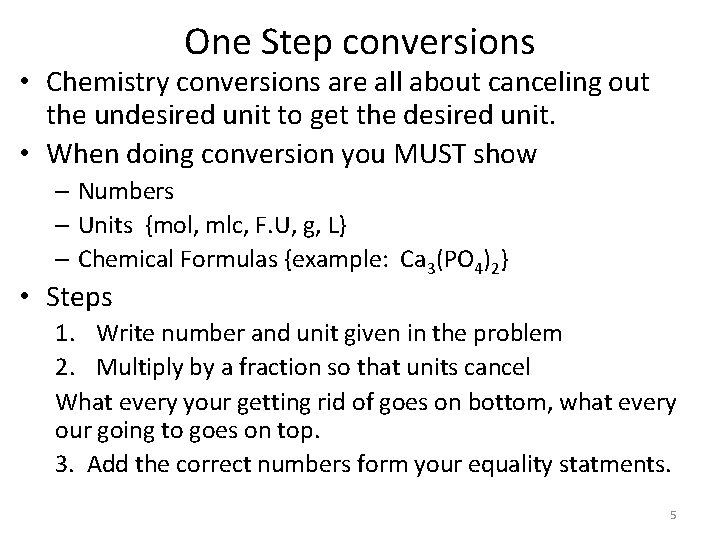One Step conversions • Chemistry conversions are all about canceling out the undesired unit to get the desired unit. • When doing conversion you MUST show – Numbers – Units {mol, mlc, F. U, g, L} – Chemical Formulas {example: Ca 3(PO 4)2} • Steps 1. Write number and unit given in the problem 2. Multiply by a fraction so that units cancel What every your getting rid of goes on bottom, what every our going to goes on top. 3. Add the correct numbers form your equality statments. 5One Step Conversions • 6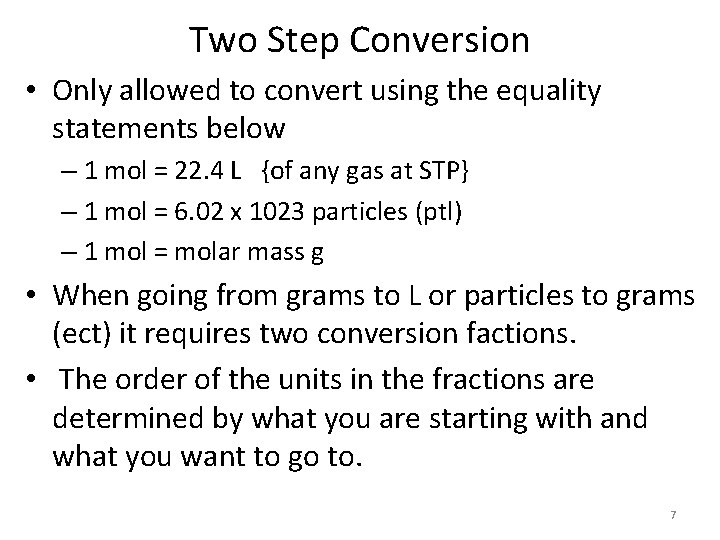Two Step Conversion • Only allowed to convert using the equality statements below – 1 mol = 22. 4 L {of any gas at STP} – 1 mol = 6. 02 x 1023 particles (ptl) – 1 mol = molar mass g • When going from grams to L or particles to grams (ect) it requires two conversion factions. • The order of the units in the fractions are determined by what you are starting with and what you want to go to. 7Two Step Conversion Raines Mole Map Each bridge is a fraction! • • Mole is always 1 Volume is always 22. 4 L Particles is always 6. 02 x 1023 ptl Mass is always molar mass of compound/element in grams. 8Two Step Conversion 9Two Step Conversion 10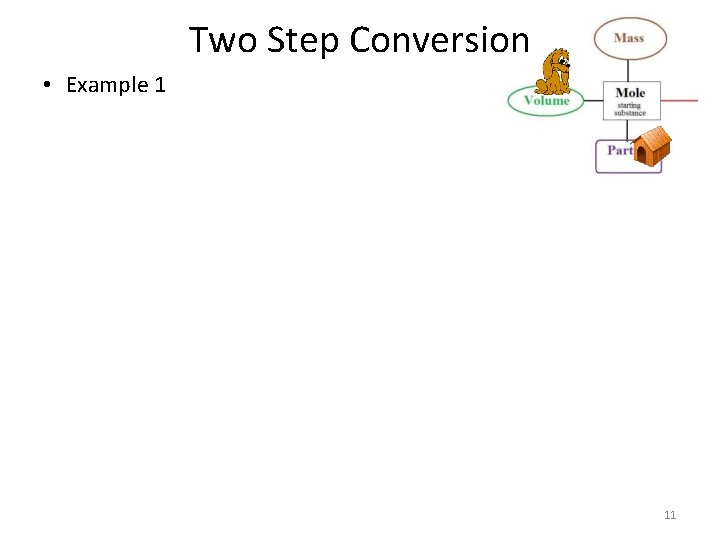Two Step Conversion • Example 1 11Text book mole map 12Percent composition • 13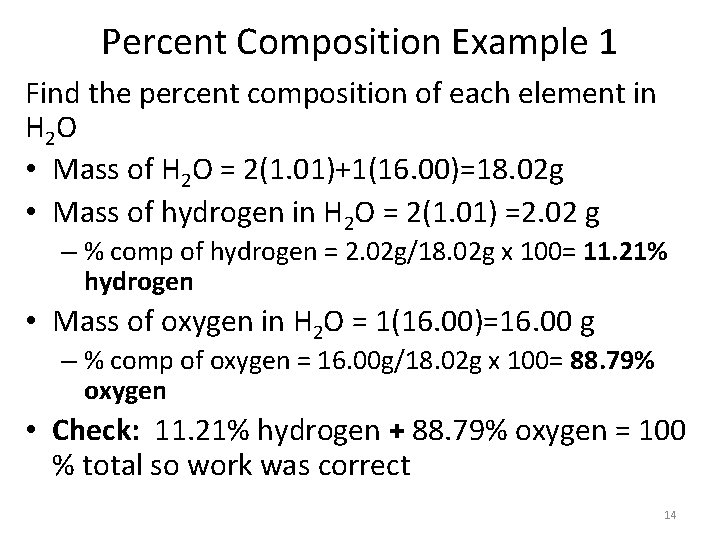Percent Composition Example 1 Find the percent composition of each element in H 2 O • Mass of H 2 O = 2(1. 01)+1(16. 00)=18. 02 g • Mass of hydrogen in H 2 O = 2(1. 01) =2. 02 g – % comp of hydrogen = 2. 02 g/18. 02 g x 100= 11. 21% hydrogen • Mass of oxygen in H 2 O = 1(16. 00)=16. 00 g – % comp of oxygen = 16. 00 g/18. 02 g x 100= 88. 79% oxygen • Check: 11. 21% hydrogen + 88. 79% oxygen = 100 % total so work was correct 14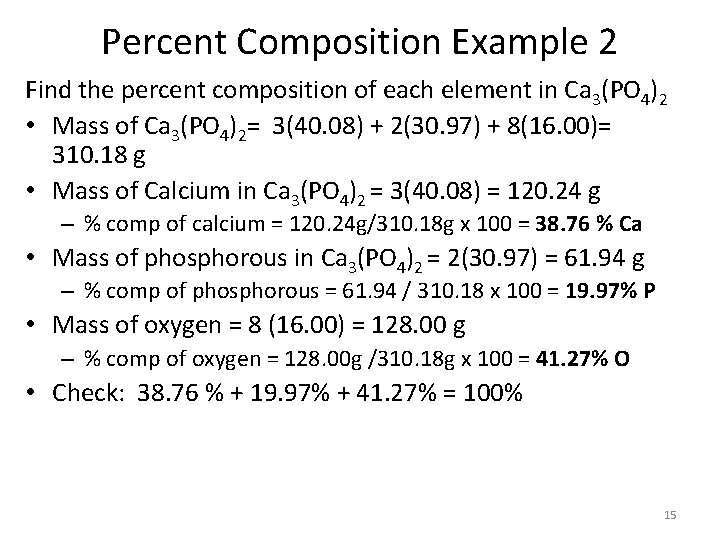Percent Composition Example 2 Find the percent composition of each element in Ca 3(PO 4)2 • Mass of Ca 3(PO 4)2= 3(40. 08) + 2(30. 97) + 8(16. 00)= 310. 18 g • Mass of Calcium in Ca 3(PO 4)2 = 3(40. 08) = 120. 24 g – % comp of calcium = 120. 24 g/310. 18 g x 100 = 38. 76 % Ca • Mass of phosphorous in Ca 3(PO 4)2 = 2(30. 97) = 61. 94 g – % comp of phosphorous = 61. 94 / 310. 18 x 100 = 19. 97% P • Mass of oxygen = 8 (16. 00) = 128. 00 g – % comp of oxygen = 128. 00 g /310. 18 g x 100 = 41. 27% O • Check: 38. 76 % + 19. 97% + 41. 27% = 100% 15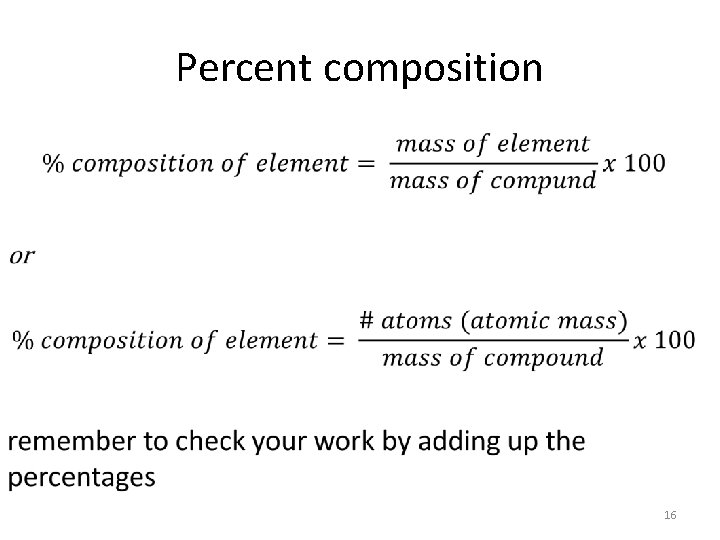Percent composition • 16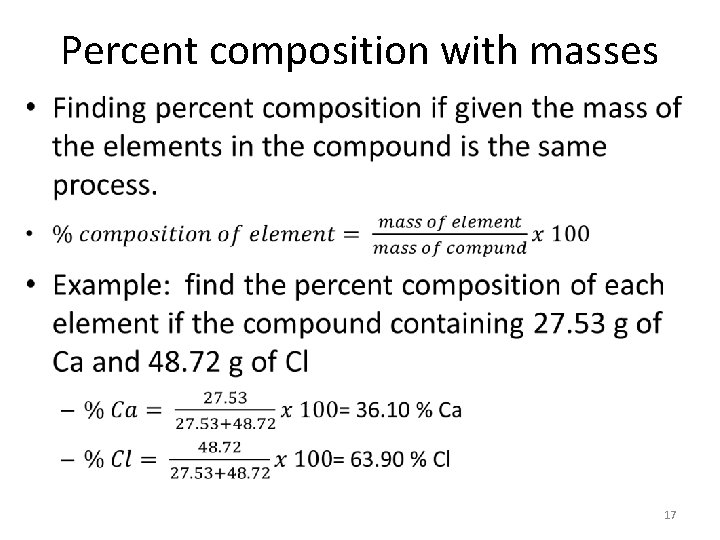Percent composition with masses • 17Using Percent Composition • If you know the percent composition (or can calculate it) it can be used to find the mass of an element in compound. • total Mass (% in decimal form) = mass of element • Example: You have a 58. 24 g sample of a substance that contains 25. 0% hydrogen. What is the mass of hydrogen in the sample – 58. 24 g (. 0250)=14. 56 g hydrogen 18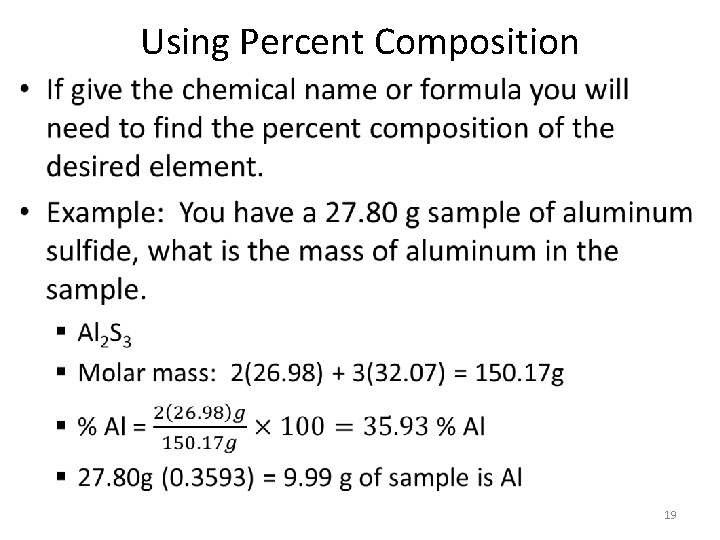Using Percent Composition • 19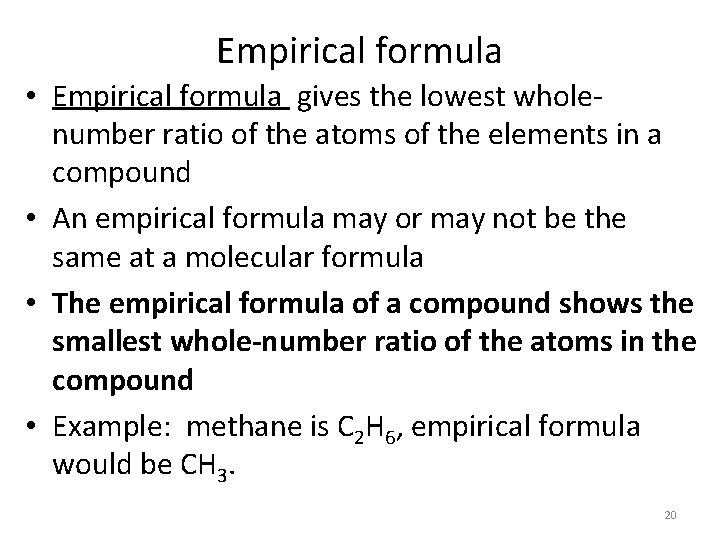Empirical formula • Empirical formula gives the lowest wholenumber ratio of the atoms of the elements in a compound • An empirical formula may or may not be the same at a molecular formula • The empirical formula of a compound shows the smallest whole-number ratio of the atoms in the compound • Example: methane is C 2 H 6, empirical formula would be CH 3. 20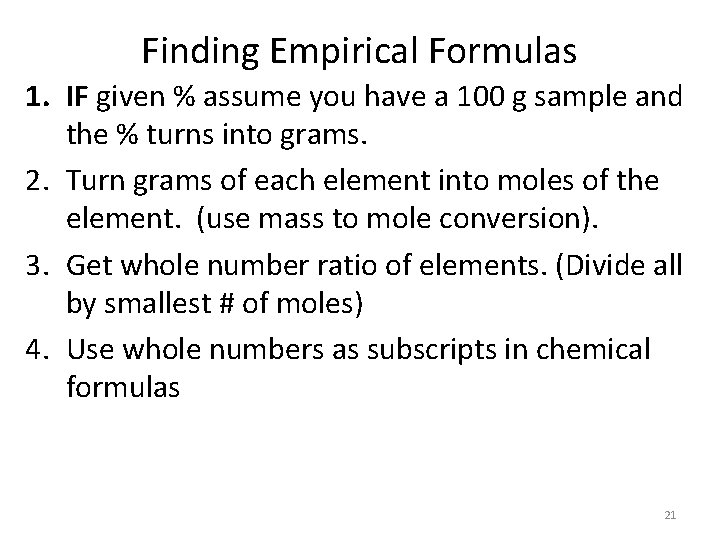Finding Empirical Formulas 1. IF given % assume you have a 100 g sample and the % turns into grams. 2. Turn grams of each element into moles of the element. (use mass to mole conversion). 3. Get whole number ratio of elements. (Divide all by smallest # of moles) 4. Use whole numbers as subscripts in chemical formulas 21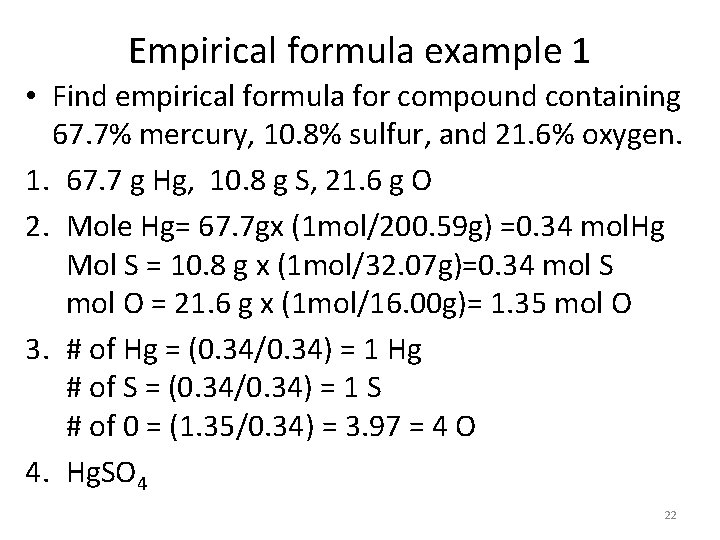Empirical formula example 1 • Find empirical formula for compound containing 67. 7% mercury, 10. 8% sulfur, and 21. 6% oxygen. 1. 67. 7 g Hg, 10. 8 g S, 21. 6 g O 2. Mole Hg= 67. 7 gx (1 mol/200. 59 g) =0. 34 mol. Hg Mol S = 10. 8 g x (1 mol/32. 07 g)=0. 34 mol S mol O = 21. 6 g x (1 mol/16. 00 g)= 1. 35 mol O 3. # of Hg = (0. 34/0. 34) = 1 Hg # of S = (0. 34/0. 34) = 1 S # of 0 = (1. 35/0. 34) = 3. 97 = 4 O 4. Hg. SO 4 22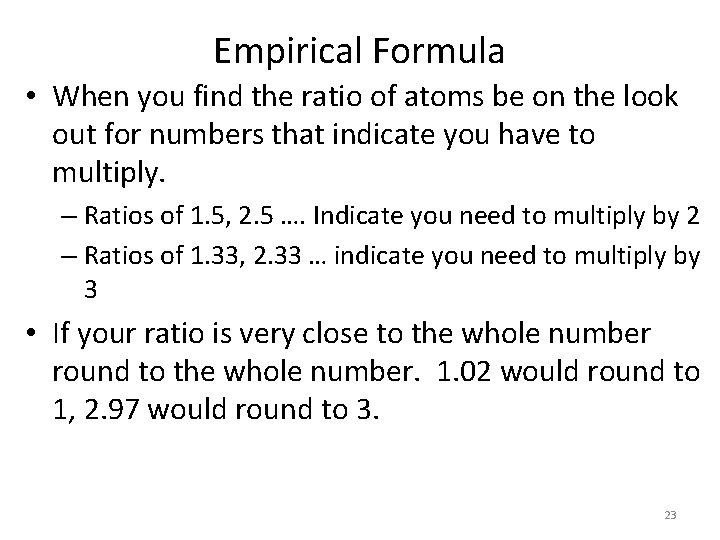Empirical Formula • When you find the ratio of atoms be on the look out for numbers that indicate you have to multiply. – Ratios of 1. 5, 2. 5 …. Indicate you need to multiply by 2 – Ratios of 1. 33, 2. 33 … indicate you need to multiply by 3 • If your ratio is very close to the whole number round to the whole number. 1. 02 would round to 1, 2. 97 would round to 3. 23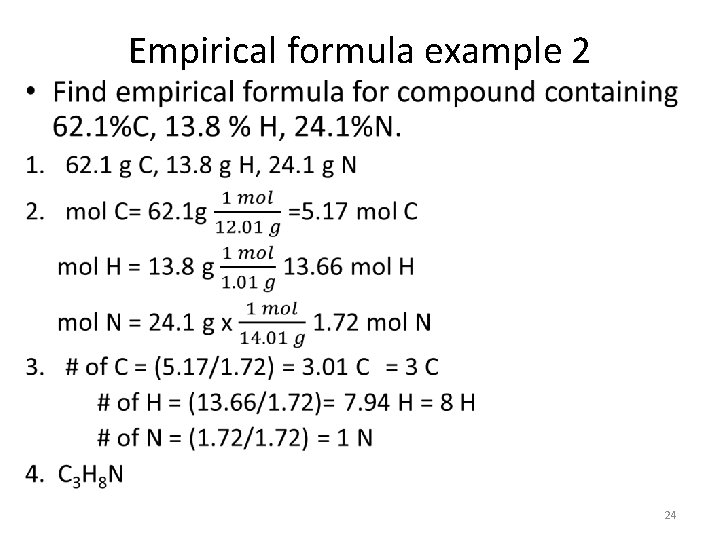Empirical formula example 2 • 24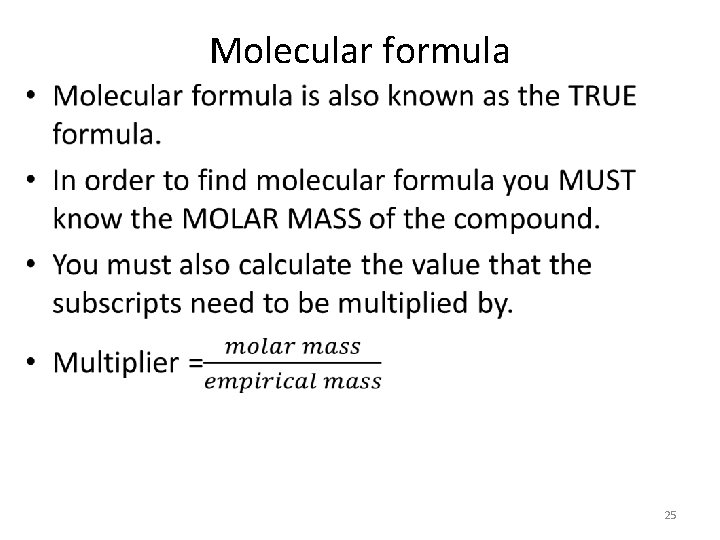Molecular formula • 25Molecular Formula Example 1 • 26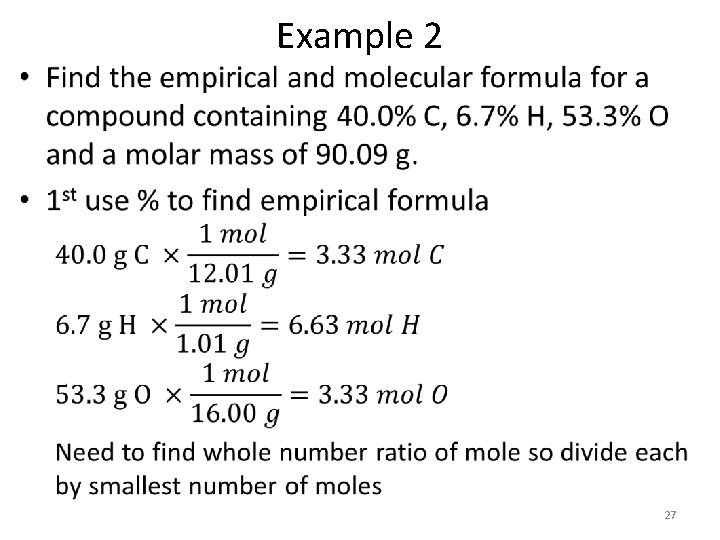• Example 2 27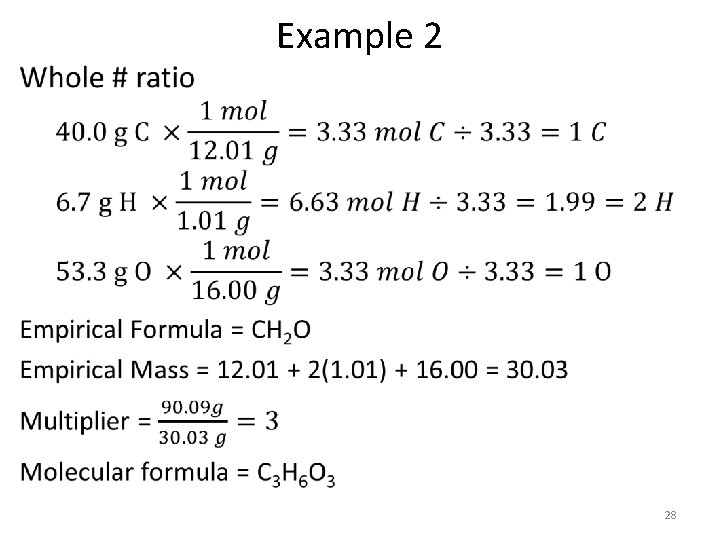• Example 2 28